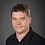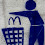## Tuesday, 12 February 2013

### Case sensitive VLOOKUP search in MS Excel

VLOOKUP is not case sensitive and when searching for 15 character Salesforce Ids, you must force Excel to match on exact case. The simplest way to return an exact case-sensitive match is by using an IF(EXACT... formula, but this will return #N/A if the first ID found is not an exact match.

The best way to enforce a case sensitive search in Excel is to use the INDEX / MATCH combo as follows.

` =VLOOKUP(FirstTab!E2,OtherTab!B:C,2,FALSE)`

then use the following:

`=INDEX(SecondTab!C:C,MATCH(TRUE,INDEX(EXACT(FirstTab!E2,SecondTab!B:B),0),0))`

Thanks to MrExcel

1.Ha, about a week later I approached this issue by calculating a second case insensitive column so I could search for duplicates - http://www.fishofprey.com/2013/02/making-salesforce-id-case-insensitive.html

2.Here are another couple of ways to search for case-sensitive duplicates in Excel.

(1) Using an in-cell formula:

=IF(B2="","",IF(SUMPRODUCT(--ISNUMBER(FIND(B2,B\$2:\$B100)))>1,"exact dupe",""))

(2) Using a conditional formatting rule:

=AND(B1<>"",SUMPRODUCT(--(EXACT(B1,\$B\$1:\$B\$100)))>1)